Math Concepts

# Tricks and Importance of Vedic Maths

13.4k views

 1 Introduction 2 Benefits of Vedic Maths 3 Addition Tricks 4 Subtraction Tricks 5 Squaring Tricks 6 Square Root Tricks 7 Multiplication Tricks 8 Conclusion 9 FAQs 10 External References

### 17 November 2020

Once the mind of a student develops an understanding of mental mathematics, he/she begins to think systematically and more creatively. In this article, we discussed some of the most basic Vedic maths tricks for beginners under different categories, with relevant examples and explanations.

Vedic Maths is a collection of techniques/sutras to solve mathematical problem sets in a fast and easy way. These tricks introduce wonderful applications of Arithmetical computation, theory of numbers, mathematical and algebraic operations, higher-level mathematics, calculus, and coordinate geometry, etc.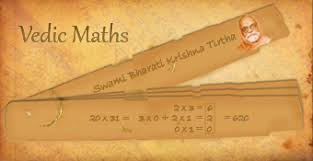It is very important to make children learn some of the Vedic maths tricks and concepts at an early stage to build a strong foundation for the child. It is one of the most refined and efficient mathematical systems possible.

Vedic maths was discovered in the mid-1900s and has certain specific principles to perform various calculations in mathematics. But the question that arises is that is mathematics only about performing calculations?

However fascinating it might be to calculate faster using Vedic mathematics tricks, it fails to make a student understand the concepts, applications, and real-life scenarios of those particular problems.

## What are the benefits of Vedic Maths?

These are the benefits of Vedic Maths:

• It helps a person to solve mathematical problems many times faster
• It helps in making intelligent decisions to both simple and complex problems
• It reduces the burden of memorizing difficult concepts
• It increases the concentration of a child and his determination to learn and develop his/her skills
• It helps in reducing silly mistakes which are often created by kids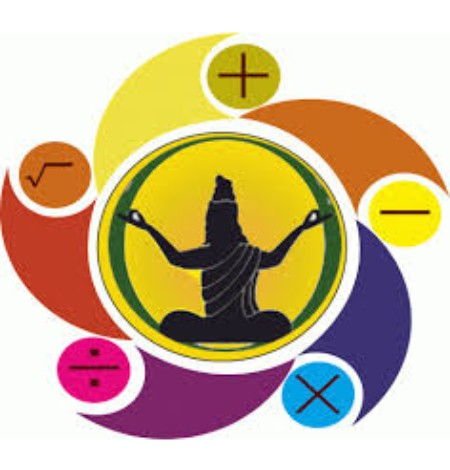## Vedic maths tricks for Addition

The addition is one of the most basic operations of Vedic mathematics. It states that,

(1) Find out the number which is closest to the 10s multiple because it is easier to add those numbers. For example,

7, 8, 9 close to 10

21, 22, 23 close to 20

67, 68, 69, are close to 70

97, 98, 99, are close to 100 ....... and so on. /p>

(2) Add the numbers which are the multiples of 10s/p>

(3) Add/Subtract the deficiency of numbers.

Let’s understand with the help of an example.

Suppose, we have to add 27 and 98.

So, Vedic maths tells us to add 30 and 100 which is 130 and then subtract (3+2) i.e. the deficiency from 130. So the result will be 125.

Similarly, if we have to add 66 and 576.

So, Vedic maths tells us to add 70 and 580 which is 650 and then subtract (4+4) i.e. the deficiency from 650. So the result will be 642.

There is yet another trick to perform addition using Vedic maths which states to add hundreds with hundreds, tens with tens and ones with ones, and so on.

For example, Suppose we have the following question, 220 + 364 + 44 + 18 = ?

Vedic maths tells us to break the numbers as per their place values. So, we will break the addition into:

200 + 300 = 500

20 + 60 +40 +10 = 130

4 + 4 +8 = 16

Repeat the process:

500 + 100 = 600

30 + 10 = 40

And at the units place we have 6.

Now perform, 600 + 40 + 6 = 646

## Vedic maths tricks for Subtraction

Vedic maths tells us to add one to the tens place to the last number and reduce the previous number by 1 and then perform subtraction.

For example,

If we have to subtract 47 from 98, then,

(1) Reduce 9 by 1 which becomes 8 and add a tens place to 8 and make it 18.

(2) Now perform 18 - 7 = 9 and 8 - 4 = 4

(3) So the answer is 49

Yet another example can be, 896 - 239,

(1) Reduce 9 by 1 which becomes 8 and covert 6 into 16.

(2) Now perform 16 - 9 = 7 and 8 - 4 = 4 and 8 - 2 = 6

(3) So the answer is 647

## Vedic maths tricks for squares

For the numbers ending with 5:

Step 1: Perform 5 × 5 = 25

Step 2: Add 1 to the previous number and the result with the previous number.

For example, in the case of the square of 85, Add 1 to 8 = 9 and multiply 9 with 8 = 72

Step 3: The result from step two will become the initial numbers of the final answer and the result of step one is the ending two numbers of the final answer. So the final answer will be 7225.

Similarly,

What is the square of 195?

Step 1: 5 × 5 = 25

Step 2: 19 × 20 = 380

Step 3: Combining the two results, which will give us 38025 which is the final answer.

### Steps for Square Roots Math tricks

For performing square roots, we will have to keep some facts in mind:-

• Squares of numbers from 1 to 9 are 1, 4, 9, 16, 25, 36, 49, 64, 81.

• Square of a number cannot end with 2, 3, 7, and 8.

• We can say that numbers ending with 2, 3, 7, and 8 cannot have a perfect square root.

• The square root of a number ending with 1 (1, 81) ends with either 1 or 9

• The square root of a number ending with 4 (4, 64) ends with either 2 or 8

• The square root of a number ending with 9 (9, 49) ends with either 3 or 7

• The square root of a number ending with 6 (16, 36) ends with either 4 or 6

• If the number is of ‘n’ digits then the square root will be ‘n/2’ OR ‘(n+1)/2’ digits.

Let us understand an example of finding a square root of 1764.

1. The number ends with 4. Since it’s a perfect square, square root will end with 2 or 8.

2. We need to find 2 perfect squares (In Multiples of 10) between which 1764 exists.
The numbers are 1600 (40) and 2500 (50).

3. Find to whom 1764 is closer. It is closer to 40. Therefore the square root is nearer to 40. Now from Step 2, possibilities are 42 or 48 out of which 42 is closer to 40.

4. Hence the square root = 42## Vedic maths tricks for Multiplication

There are a number of techniques to perform various types of multiplication calculations using Vedic maths tricks. Some of the most useful and the easiest ones are mentioned below:-

The trick of 11:

Step 1:- Divide the number into two parts

Step 2:- Add the two parts which will form the middle number

Let us understand this with the help of an example which will clear the doubts.

Let us say that we have to multiply 32 with 11.

Step 1: Divide 32 into 3 and 2

Step 2: The middle will be 3 + 2 = 5

So our answer to 32 × 11 would be 352.

Similarly, 75 × 11 = 7, 7 + 5, 5. Because 7+5 = 12, we will carry 1 to the previous digit and our final answer would be 825.

Multiplying numbers which are close to powers of 10:

It would be easy to directly jump into the example and understand this concept through it. Suppose, we have 2 questions:

1. Multiply 99 × 97

2. Multiply 103 × 105

Our approach should be something as shown in the picture.

Step 1: Find by how much the number is more or less than the power of 100 and write it on the right side of the vertical line.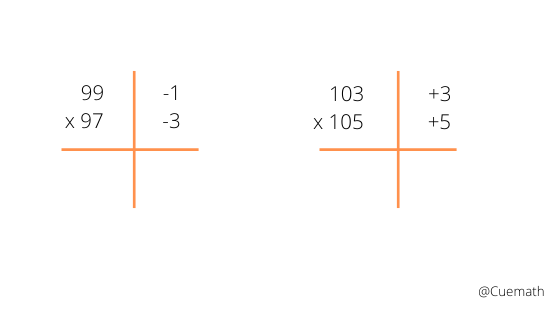Step 2: Either cross subtract or cross add. This will form the first part of the final answer.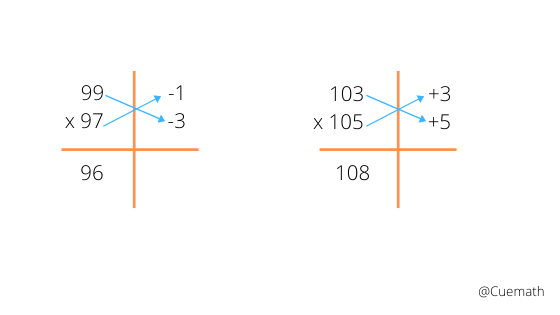Step 3: Multiply the right side of the vertical line and this shall form the right side of the result.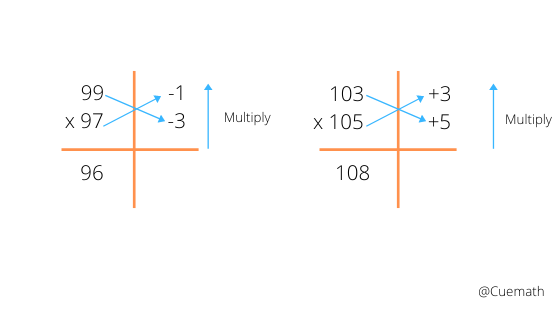Similarly, suppose we have to find 996 × 997. Our approach will be as shown in the picture below.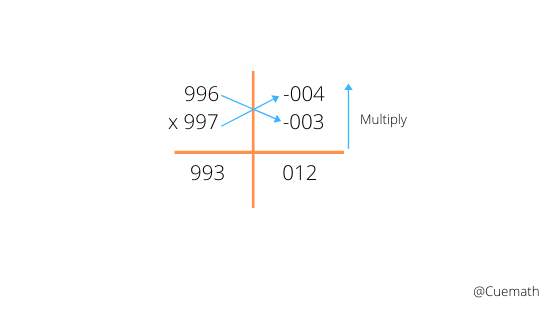Multiplying 2 digit numbers:

This would again be simple if followed by a step approach through what is displayed in the picture. Suppose, we have to multiply 31 × 12. We have to follow the vertical and crosswise sutra. Our approach would be:-

Step 1: Multiplying vertically the units place.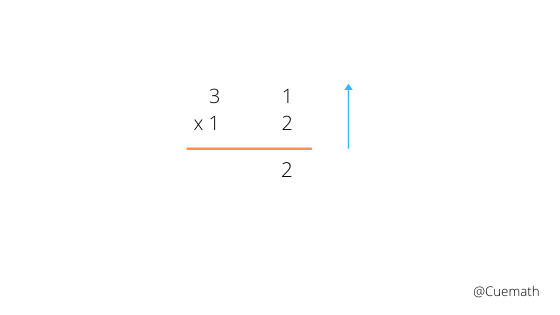Step 2: Multiplying in a cross pattern and adding the results. For example, 3 × 2 = 6 and 1 × 1 = 1 and 6 + 1 = 7.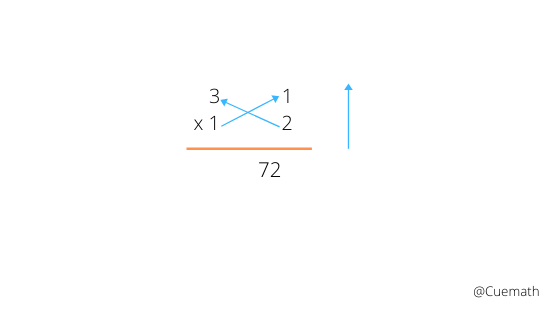Step 3: Multiplying the tens digit vertically.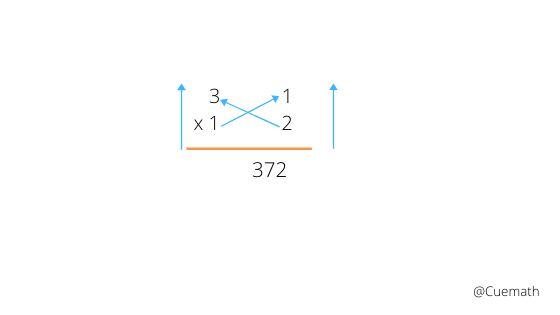Similarly, if we have to find 12 × 34, our approach will be as shown below:

Firstly, multiply 2 × 4 = 8

Then, (3 × 2) + (4 × 1) = 10. The zero remains and 1 is carried to the left.

Finally, (3 × 1) = 3. Add to it the carried number so that it becomes, (3 + 1) = 4.

Our final answer would be 408.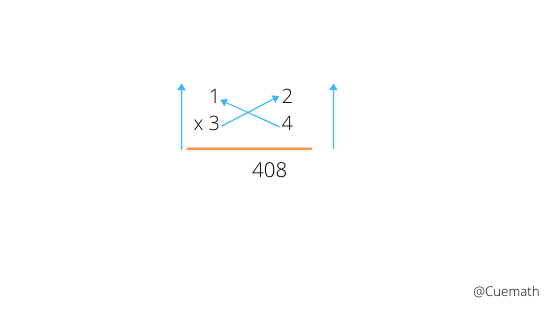## Conclusion

Vedic Maths just showcases a process to do things faster. It does not teach a child the underlying philosophy or the background of the problem set given. Calculating faster is of no use if we fail to understand the meaning or the learning behind the problem set.

These tricks can do wonders only if used properly after imbibing a proper learning experience. Practice makes a man perfect but Learning makes a man capable. Therefore, make Vedic Maths a habit only after understanding its nuances.

Cuemath comes up with regular articles on varied topics, providing positive thinking and direction on different issues faced by children and parents. Comment in the box below any topic, doubts, or other feedback. We will surely research and reach out to you soon.

Cuemath, a student-friendly mathematics and coding platform, conducts regular Online Live Classes for academics and skill-development, and their Mental Math App, on both iOS and Android, is a one-stop solution for kids to develop multiple skills. Understand the Cuemath Fee structure and sign up for a free trial.

## What are some applications of Vedic maths?

Starting from arithmetic operations, geometry, trigonometry, integral, and calculus there are endless possibilities in applications of Vedic maths.

## Who found Vedic Maths?

The method of Vedic Maths is found by the ancient sages and rediscovered by

monk Bharati Krishna Tirtha who wrote a book named,

Vedic Mathematics and first published in 1965. It contains a list of mathematical techniques, which the author claimed were retrieved from the Vedas and supposedly contained all mathematical knowledge.

## External References

Wikipedia.org - Vedic Mathematics

Advantages of Vedic Mathematics | Vedic Mathematics

Related Articles
GIVE YOUR CHILD THE CUEMATH EDGE
Access Personalised Math learning through interactive worksheets, gamified concepts and grade-wise courses